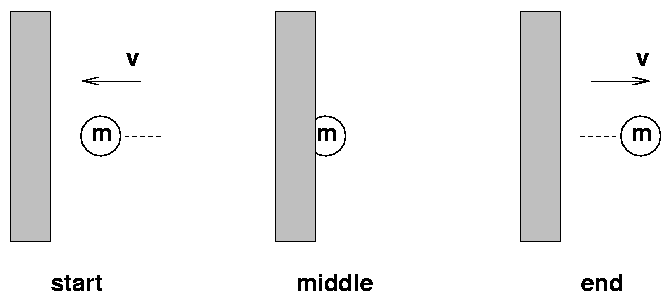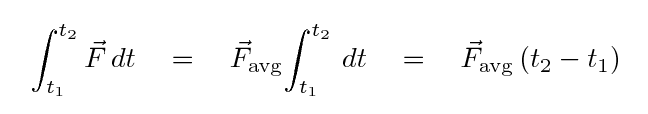Copyright © Michael Richmond. This work is licensed under a Creative Commons License.

# Impulse on a tennis ballA tennis ball of mass m = 0.03 kg flies towards a wall with velocity v = 20 m/s west. It hits the wall, stops momentarily, and then bounces back with the same speed in the opposite direction.

1. What is the ball's initial momentum?
2. What is the ball's final momentum?
3. What is the impulse, defined as the change in momentum
```
impulse  =   (final momentum)  -  (initial momentum)
```
4. We can simplify things a bit by defining an average force over the course of the impact.If the ball is in contact with the wall for about Δt = 0.03 s, then what is the average force on the ball during that time?Copyright © Michael Richmond. This work is licensed under a Creative Commons License.